# Texas Go Math Grade 7 Module 13 Answer Key Taxes, Interest, and Incentives

Refer to our Texas Go Math Grade 7 Answer Key Pdf to score good marks in the exams. Test yourself by practicing the problems from Texas Go Math Grade 7 Module 13 Answer Key Taxes, Interest, and Incentives.

## Texas Go Math Grade 7 Module 13 Answer Key Taxes, Interest, and Incentives

Texas Go Math Grade 7 Module 13 Are You Ready? Answer Key

Write each percent as a decimal.

Question 1.
45% ______
Write the percent as the sum of 1 whole and a percent remainder.
100% – 55% = $$\frac{100}{100}$$ – $$\frac{55}{100}$$ Write the percents as fractions.
= 1 – 0.55 Write the fractions as decimals.
= 0.45

Question 2.
91% ______
Write the percent as the sum of 1 whole and a percent remainder.
100% – 9% = $$\frac{100}{100}$$ – $$\frac{9}{100}$$ Write the percents as fractions.
= 1 – 0.09 Write the fractions as decimals.
= 0.91

Question 3.
8%
Write the percent as the sum of 1 whole and a percent remainder.
100% – 92% = $$\frac{100}{100}$$ – $$\frac{92}{100}$$ Write the percents as fractions
= 1 – 0.92 Write the fractions as decimals
= 0.08

Question 4.
111%
Write the percent as the sum of 1. whole and a percent remainder.
100% + 11% = $$\frac{100}{100}$$ – $$\frac{11}{100}$$ Write the percents as fractions.
= 1 + 0.11 Write the fractions as decimals.
= 1.11

Write each decimal as a percent.

Question 5.
0.79 _______
Write the decimal as the sum of 1 whole and a decimal remainder.
1 – 0.21 = $$\frac{100}{100}$$ – $$\frac{21}{100}$$ Write the decimals as fractions.
= 100% – 21% Write the fractions as percents.
= 79%

Question 6.
0.8 ________
Write the decimal as the sum of 1 whole and a decimal remainder.
1 – 0.21 = $$\frac{100}{100}$$ – $$\frac{20}{100}$$ Write the decimals as fractions.
= 100% – 20% Write the fractions as percents.
= 80%

Question 7.
0.05 ____
Write the decimal as the sum of 1 whole and a decimal remainder.
1 – 0.95 = $$\frac{100}{100}$$ – $$\frac{95}{100}$$ Write the decimals as fractions.
= 100% – 95% Write the fractions as percents.
= 5%

Question 8.
1.98
Write the decimal as the sum of 1 whole and a decimal remainder.
1 + 0.98 = $$\frac{100}{100}$$ + $$\frac{98}{100}$$ Write the decimals as fractions.
= 100% + 98% Write the fractions as percents.
= 198%

Find each sum or difference.

Question 9.
11.9 – 7.6 _________4.3

Question 10.
24.1 – 9.25 ______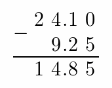14.85

Question 11.
45 – 10.6 ________34.4

Question 12.
6.04 – 3.5 _________2.54

Question 13.
5.17 – 5.09 ______0.08

Question 14.
100 – 3.77 _______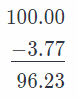96.23

Multiply.

Question 15.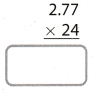Multiply as whole numbers.
Count the total number of decimal places in the both factors, and put the same number of decimals in the product.66.48

Question 16.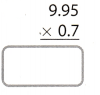Multiply as whole numbers.
Count the total number of decimal places in the both factors, and put the same number of decimals in the product.6.965

Question 17.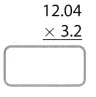Multiply as whole numbers.
Count the total number of decimal places in the both factors, and put the same number of decimals in the product.38.528

Question 18.Multiply as whole numbers.
Count the total number of decimal places in the both factors, and put the same number of decimals in the product.27.648

Texas Go Math Grade 7 Module 13 Reading Start-Up Answer Key

Visualize Vocabulary

Use the ✓ words to complete the graphic. You will put a different word in each box.Understand Vocabulary

Complete each sentence using the preview words.

Question 1.
The amount of money earned by bank customers based on the amount of principal in their savings account is _____